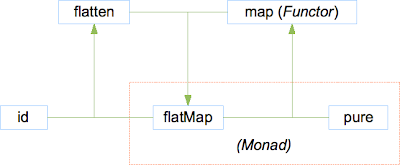Wednesday, October 29, 2008

Some weeks ago, I spent a couple of days working through some Haskell with a colleague of mine. This is some of the stuff we went through.

1. Haskell exercises for beginners to get back in the swing of things.

2. Write implementations for:
`mflatten :: Maybe (Maybe a) -> Maybe ammap     :: (a -> b) -> Maybe a -> Maybe blFlatMap :: [] a -> (a -> [] b) -> [] bmFlatMap :: Maybe a -> (a -> Maybe b) -> Maybe b`

3. Observe `lFlatMap` and `mFlatMap` can be written with the same pattern. How can we reduce the "duplication"?

4. Derive `flatten` from `flatMap`:
`class FlatMap f where  flatMap :: f a -> (a -> f b) -> f bflatten' :: (FlatMap f) => f (f a) -> f aflatten' = error "todo"`

5. Given `pure`:
`class Pure p where  pure :: a -> p a`
and `flatMap`, derive `map`:
`map' :: (FlatMap f, Pure f) => f a -> (a -> b) -> f bmap' t f = error "todo"`

6. Let's give the combination of `flatMap` and `pure` a name, perhaps `Monad`:
`class Monad' m where  flatMap :: m a -> (a -> m b) -> m b  pure    :: a -> m a`
A diagram to describe the relationships between our functions so far:7. Write the following functions:
`liftM0    :: (Monad' m) => a -> m aliftM1    :: (Monad' m) => (a -> b) -> m a -> m bliftM2    :: (Monad' m) => (a -> b -> c) -> m a -> m b -> m cliftM3    :: (Monad' m) => (a -> b -> c -> d) -> m a -> m b -> m c -> m dap        :: (Monad' m) => m (a -> b) -> m a -> m bsequence' :: (Monad' m) => [m a] -> m [a]mapM'     :: (Monad' m) => (a -> m b) -> [a] -> m [b]`

Observe:
• `liftM0` and `liftM1` are just `pure` and `map` respectively

• `liftMn` for n >= 2 can be written as:

• n x `flatMap` + pure

• (n - 1) x `flatMap` + `map`

• `liftM`(n - 1) + `flatMap`

• `liftM`(n - 1) + `ap`

• `sequence'` and `mapM'` can each be written in terms of the other

8. Finally, note the Monad laws.

Step 2 Notes
I found it natural to write:
`lFlatMap xs f = concat \$ map f xsmFlatMap Nothing _  = NothingmFlatMap (Just x) f = f x`
However, given the similar type signatures, I can instead write:
`mFlatMap xs f = mflatten \$ mmap f xs`

Step 3 Notes
After some reflection I realised it is important is to ignore the function names and implementations. I had internalised the concept of `map` as a minor generalisation of `List.map` i.e. traversing across elements in a 'container' and applying the same function to each one, which didn't quite fit with `Maybe`.

The key is simply the function signatures. If I have some functions `f` and `g`, such that
`f :: (a -> b) -> t a -> t bg :: t (t b) -> t b`
then I can automatically construct a function `h`
`h :: t a -> (a -> t b) -> t b`
where `h` is simply the composition of `g` with `f` and nothing more can be said about it.

It does not matter that `f` is called `map` for `List` and it is not helpful to compare implementations of `f` for two different types `t`, looking for similarities beyond the type signature.

For me this is a case where attaching a meaning to a name impeded the process of abstraction.

Quiz said...

"It does not matter that f is called map for List and it is not helpful to compare implementations of f for two different types t, looking for similarities beyond the type signature."

You are right that those things don't matter, but one needs more than type signatures: one needs laws.

You need for f (i.e. fmap or liftM1) to obey the functor laws: fmap id = id, and fmap f . fmap g = fmap (f . g).